# The perimeter

The perimeter of the triangle is 24, the sides are integers and form an arithmetic sequence. Specify the side sizes of this triangle.

a1 =  5
b1 =  8
c1 =  11
a2 =  6
b2 =  8
c2 =  10
a3 =  7
b3 =  8
c3 =  9

### Step-by-step explanation:

${b}_{1}={a}_{1}+{x}_{1}=5+3=8$
${c}_{1}={b}_{1}+{x}_{1}=8+3=11$
${b}_{2}={a}_{2}+{x}_{2}=6+2=8$
${c}_{2}={b}_{2}+{x}_{2}=8+2=10$
${b}_{3}={a}_{3}+{x}_{3}=7+1=8$
${c}_{3}={b}_{3}+{x}_{3}=8+1=9$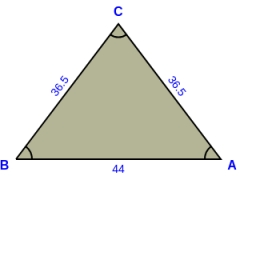Did you find an error or inaccuracy? Feel free to write us. Thank you!Tips to related online calculators
Do you solve Diofant problems and looking for a calculator of Diofant integer equations?

#### You need to know the following knowledge to solve this word math problem:

We encourage you to watch this tutorial video on this math problem:

## Related math problems and questions:

• The sidesThe sides of a right triangle form an arithmetic sequence. The hypotenuse is 24 cm long. Determine the remaining sides of the triangle.
• AP RT triangleThe length of the sides of a right triangle forms an arithmetic progression, and the longer leg is 24 cm long. What are the perimeter and area?
• Find the 21Find the sum of the six terms of the finite geometric sequence 96, -48, 24, -12
• Angles of a hexagon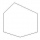Find the interior angles of a hexagon if the sizes of the angles form an arithmetic sequence, and the smallest angle is 70°.
• Rectangular triangleThe lengths of the rectangular triangle sides with a longer leg of 12 cm form an arithmetic sequence. What is the area of the triangle?
• Integer sidesA right triangle with an integer length of two sides has one leg √11 long. How much is its longest side?
• Medians in RT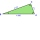The rectangular triangle ABC has a length of 10 cm and 24 cm. Points P, Q, R are the centers of the sides of this triangle. The perimeter of the PQR triangle is:
• Right-angled triangle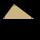Determine the content of a right triangle whose side lengths form successive members of an arithmetic progression and the radius of the circle described by the triangle is 5 cm.
• Geometric sequence 4It is given geometric sequence a3 = 7 and a12 = 3. Calculate s23 (= sum of the first 23 members of the sequence).
• Sides of triangleTriangle has circumference 42 cm. Side a is 2 times shorter than side b and sice c is 2 cm longer than side a. Determine the sizes of sides of a triangle.
• Isosceles triangle 9Given an isosceles triangle ABC where AB= AC. The perimeter is 64cm, and the altitude is 24cm. Find the area of the isosceles triangle.
• Angles in a triangleThe angles of the triangle ABC make an arithmetic sequence with the largest angle γ=83°. What sizes have other angles in a triangle?
• The sides 3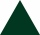The sides of an equilateral triangle are 9.4 cm, correct to the nearest one decimal place. Work out the upper bound of the side of this triangle.
• RectangleThe perimeter of the rectangle is 22 cm and content area 30 cm2. Determine its dimensions, if the length of the sides of the rectangle in centimeters is expressed by integers.
• Trapezoid - hard exampleBase of the trapezoid are: 24, 16 cm. Diagonal 22, 26 cm. Calculate its area and perimeter.
• GP - three membersThe second and third of a geometric progression are 24 and 12(c+1), respectively, given that the sum of the first three terms of progression is 76. determine the value of c.
• Rhombus diagonalsIn the rhombus ABCD are given the sizes of diagonals e = 24 cm; f = 10 cm. Calculate the side length of the diamond and the size of the angles, calculate the content of the diamond